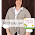## Monday, March 17, 2014

### Monday Math Literature Volume 35

If you missed last week's post about some great primary shape books, check it out here!

Today I want to share with you some great math literature books about fractions.  Both of these books were written by David Adler.  He is the author of Time Zones, a book I reviewed a few weeks ago as well as the Cam Jansenseries of books and several other math literature stories.

Working With FractionsThis story gives a comprehensive look at fractions.  I have used it in grades 3-6.  I love how it gives many different examples of where fractions are found including examples of area and set models.  It does not go into number line models but shows many examples of fractions in the area and set context.  It also goes into details about fraction equivalency and magnitude.  It has a nice explanation of how multiplying by a fraction equal to one produces equivalent fractions.  It ends with some basic ideas around fraction addition and subtraction.  I like this book as a way to wrap up a unit on fractions or part way through a unit to review what students have learned and foreshadow what they will be learning next.

Fractions, Decimals, and PercentsI love how this story explores fractions, decimals and percents in the context of a county fair.  It is a fun way to see the connection between these different types of numbers.  I have used this book in grades 4-6.  In fourth grade I spend a lot of time helping kids make the connection between fractions, decimals and money.  I use this book as a follow up to my fraction, decimal and money task cards.  In grades 5 and 6 percents play a bigger role in the curriculum and this book helps kids connect back to what they already know about fractions and decimals.

Looking for other books on fractions?
Here is one that is a great introduction to fractions for grade 1 and 2
Here is a great one for grades 5-7 on multiplying and dividing fractions
Here is a Hershey chocolate themed one for grades 4 and 5
Here is another great one for primary students!

#### 1 comment:

1.Thanks for sharing these awesome suggestions! We just started our fractions units! It is one of my favorite units to teach! I am your newest follower via bloglovin!
Michelle
A New Day of Learning